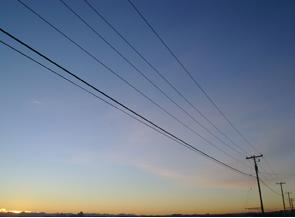# Minimising Line Losses in RE Systems

In most renewable energy systems low voltage DC (direct current) electricity is generated. When this is from a wind turbine generator or a hydro electricity generator there is usually quite a distance between the location of the generator and the battery bank or power inverter.If the wire (cable) taking this electricity is not thick enough you may potentially lose vast amounts of expensively generated power in resistive line losses – basically speaking, you will be heating the wire rather than charging your batteries. However, with the recent jump in the price of copper, if you choose wire which is unnecessarily thick, the extra costs incurred will seriously get in the way of your generating free (not that such a thing exists) electricity.

In an ideal situation line losses should be kept at or below 10% – i.e. no more than 10% of the power you generate should be lost in the wires you use to transmit it to your battery bank or inverter.

### What You Need to Know to Calculate Line Losses

Calculating your likely line losses is quite a simple process requiring no more than a standard calculator and a little knowledge – supplied here.

NOTEIf you are not interested in the mathematics then scroll down to the final equations so you can start making some calculations for your system.
NEW You can also click here to go to our new Line Losses Calculator which will automatically calculate the size of wire you require for your system, or calculate the line losses for a given wire size.

The one constant you need is the resistivity of copper (rho) = 0.000000017 Ohms per metre at 20 degrees Celcius.

You should know the charging voltage (peak) and current (peak) of your generator. The charging voltage is always higher than the rated voltage of a generator – e.g. a 12 Volt solar panel will actually have an open circuit voltage of at least 18 Volts. You need to use the higher value in your calculations.

The cross-sectional area of your wire is given by multiplying the radius (half the diameter) of the wire squared by PI (3.14159).

If the wire is made up of multple strands then the cross-sectional area is given by squaring the radius of one strand and multiplying it by PI and then by the number of strands.

Once you know these values you are ready to calculate the resistance of your power lines and therefore your power losses.

### Calculating Line Losses

The resistance of a length of wire is equal to 1,000,000 multiplied by the length of the wire (in metres) multiplied by rho and then divided by the cross sectional area of the wire (in square mm). Note that 1,000,000 square mm is equal to one square metre – hence the factor of 1,000,000 in the equation.

For example:
A wire 200 metres long with a cross sectional area of 2.25 square mm has a resistance of:

(1,000,000 * length * 0.000000017) / 2.25 = 1.51 ohms

The power loss is given by the square of the current in amps multiplied by the resistance of the wire, so with a 30 amp peak power output wind turbine generator and the example wire above, the power loss is:

30 * 30 * 1.51 = 1,359 Watts

If the example wind turbine has a charging voltage of 65 volts (typical of a 48 Volt rated wind turbine) then the peak power output of this wind turbine would be equal to the charging voltage multiplied by the current = 65 x 30 = 1,950 Watts.

Therefore of the 1.95kW generated by the wind turbine, 1.36kW would be lost as line losses = 70%!

100 * 1.36/1.95 = 70%

### Using the Equation to Select wire for Your System

All the mathematics above can be simplified into one easy (relatively) equation:

Power Loss (%) =
 1.7 * Current * Wire Length Charging Voltage * Wire Area

In order to choose wire for your own renewable energy system you just need to choose the amount of line losses you are willing to accept (e.g. 10%), feed the numbers into the equation below, and out comes the minimum cross-sectional area of wire you will need.

Wire X-Sectional Area =
 1.7 * Current * Wire Length Charging Voltage * Power Loss %

So with the example 48V wind turbine generator which had a 65 Volt charging voltage and 30 amp maximum current, a 200 metre run of wire required, and no more than 10% line losses acceptable – wire with a cross sectional area of 15.7 square mm is required:

(1.7 * 30 * 200) / (65 * 10) = 15.7 sq.mm

Looking at the wires available on the market around this size there is 6 AWG (13.29 sq.mm) and 5 AWG (16.76 sq.mm). To keep line losses under 10% the 5 AWG would have to be selected giving 9.3% resistive line losses in this example.